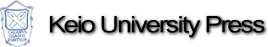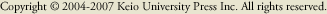MATHEMATICAL FOUNDATIONS OF MEASUREMENT THEORYMATHEMATICAL FOUNDATIONS OF MEASUREMENT THEORY
 (front cover)The mathematical foundations of measurement theory will be proposed in this book.  As it is usually said that there is no science without measurement , the concept of "measurement" is certainly the most fundamental in science. In this book, the measurement theory ( proposed by the author recently) is comprehensively introduced to graduate students chiefly. The measurement theory has several aspects, that is,  1.the mathematical representation of "the mechanical world view"  2.the axiomatic formulation of system theory and statistics  3.the algebraic abstraction of quantum mechanics  4.the most fundamental theory of engineering  5.the mathematical representation of philosophical epistemology In the most part of this book, the above 2. and 3. are mainly and mathematically treated.  All people that are involved in science may be recommended to read this book.